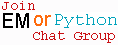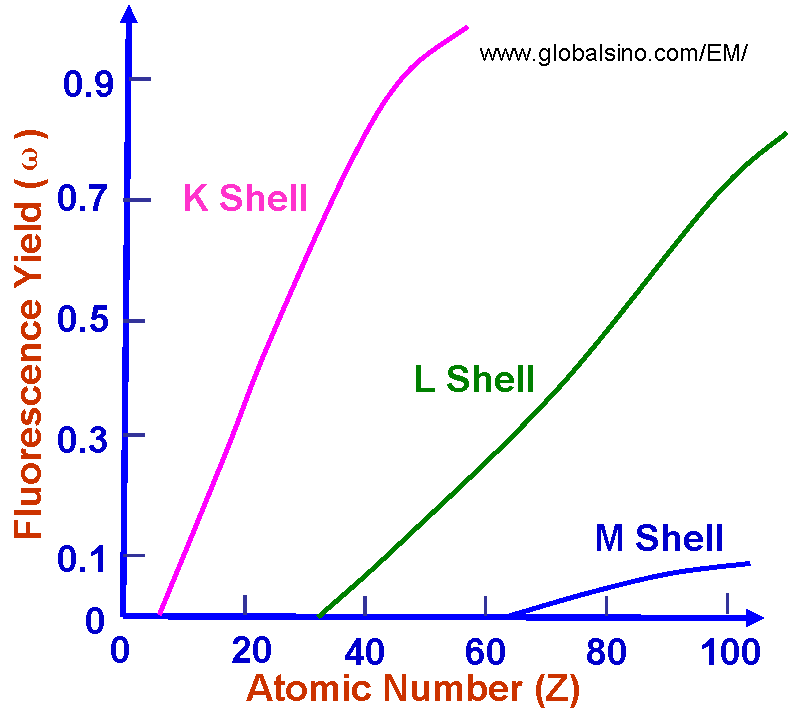X-Ray (Fluorescence) Yield/Weight
- Practical Electron Microscopy and Database -
- An Online Book -Microanalysis | EM Book                                                                   http://www.globalsino.com/EM/

For a K-shell ionization, the X-ray yield ϖK is defined as the fraction of atomic decays by emitting a K-shell X-ray. The rate of X-ray emission is evaluated for an electric dipole transition between the two atomic states of the atom, |i> and |j>, and is the ratio of the X-ray rate to the total rate, which includes the X-ray rate and Auger electron rate. For a K-shell emission, ϖK empirically depends on atomic number, Z, given by,

ϖK = Z4/(106+Z4) ----------------------------- 

Therefore, heavy elements tend to emit X-rays and light elements tend to emit Auger electrons.

Figure 4637a shows X-ray (fluorescence) yield ω as a function of atomic number for electron ionization within the K, L, and M electron shells. The yield is defined by emitted photons per ionization, which is the fraction of ionizations that results in X-ray emission. For each shell, we have ωK > ωL > ωM, where ωK, ωL, and ωM are yields for K, L, and M shells, respectively. For atoms with low atomic numbers, e.g. Z < 26, there are no ωL and ωM yields because there are less electrons. Detailed information on yields, sometimes called weights and refered to intensities, can be found in the Section Photon Energies and Relative Intensities of K-, L-, and M-Shell Lines.Figure 4637a. X-ray (fluorescence) yield ω as a function of atomic number for electron ionization within the K, L, and M electron shells.

As an example, Figure 4637b shows the X-ray and Auger electron yields per K vacancy as a function of atomic number as well as from all vacancies generated by accelerated incident electrons. For low-Z material Auger emission is dominant, while for high-Z material X-ray emission dominates. The two emission probabilities are approximately equal at Z ≈ 30 for K shell ionization.Figure Figure 4637b. X-ray and Auger electron yields per K vacancy as a function of atomic number.

The relationship between the yields of K X-ray lines (ωK) and K Auger electrons (αK) per K vacancy is given by,
ωK + αK = 1 ------------------------------------- [4637a]
And, the relationship between the yields of all X-ray lines (ωall) and all Auger electrons (αall) per vacancy is given by,
ωall + αall = 1 ------------------------------------- [4637b]

Furthermore, X-ray emission also depends on the energy of incident beam. For incident electrons at low energies, it is very important to know that a useful X-ray signal can only be generated with a beam energy which is at least 1.3 x the ionization energy for the relevant characteristic X-rays. X-ray yields, in this condition, are also relative low and depend strongly on overvoltage  (especially when U<3) and the X-ray signals are relatively weak. For effective generation of X-ray by ionization processed by electron interaction, the overvoltage U should be greater than 1.3 because the X-ray peak to background ratio (P/B) of X-ray signal is reduced substantially as E0 (energy of incident beam) approaches Ei (ionization energy). It is necessary to mention that the low energy X-ray lines, e.g. Cu(L) at 0.93 keV instead of Cu(K) at 8.04 keV, are used at low accelerating voltage of incident electrons.

 ;